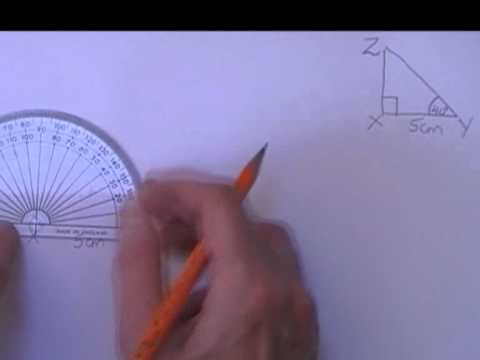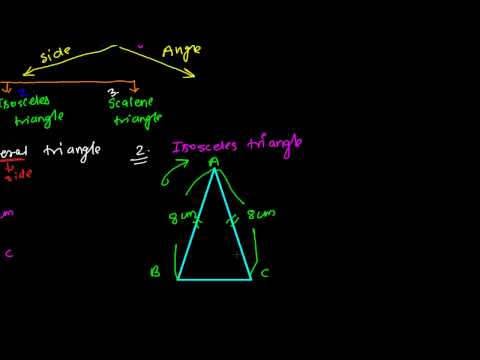## Trigonometry

Subject: Optional Maths

Find Your Query

#### Overview

This note gives information about Triangles.And triangle on the basis of sides, angles, even it gives detail of right-angled triangle.

### Triangles

A triangle is a geometrically closed figure which is bounded by three lines and angles. It is categorized into two types as sides and angles.

#### Triangles on the basis of sides

We can categorize the triangle into following 3 types on the basis of sides:

• Equilateral triangle
A triangle having all sides equal is known as an equilateral triangle. In figure (i) we have observed a triangle with all 3 sides are equal.

• Isosceles triangle
A triangle having any two sides equal is known as an isosceles triangle. In figure (ii), we can see a triangle with two sides equal.

• Scalene triangle
A triangle having none of the sides equal is known as an isosceles triangle. In figure (iii), we can see a triangle with no sides equal to each other.

#### Triangle on the basis of angle

We can categorize the triangle into following 3 types on the basis of angle:• Acute angled triangle
A triangle having an angle less than 90 degrees is known as acute angled triangle. In figure (i), we have seen a triangle having less than 90.

• Obtuse angle triangle
A triangle having an angle more than 90 degrees is known as obtuse angled triangle. In figure (ii), one of the angles is more than 90 degree.

• Right angle triangle
A triangle having an angle exactly 90 degree is known as right angled triangle. In figure (iii), one of angle is 90.

#### Reference angle

A right-angled triangle which is taken into consideration is known as the reference angle. For example, Assuming $\angle$ EFG as the reference angle. where angle E is known as remaining angle. So,opposite to the right angle is known as hypotenuse and it is denoted by h. The side opposite to the reference angle, the side is called perpendicular which is denoted by p and remaining side are known as the base and it is denoted by b ,

Here,

EF = hypotenuse(h)

EG=Perpendicular (p)

FG= base(b)

In trigonometry, most of the reference angles are represent by verious Greek alphabets. Some of them are given below:

 alpha $\alpha$ beta $\beta$ gamma $\gamma$ delta $\delta$

We normally use these symbols for representing reference angles.

For example,

Here, $\triangle$ EFG is a right angled triangle, right angled at F.

Let, ∠GEF = θ be the reference angles .

Then, side EG = hypotenuse ( h ) [$\therefore$It is opposite to right angle ]

side EF = perpendicular ( p ) [$\therefore$It is opposite to reference angle ]

side FG = base ( b ) [$\therefore$It is side opposite to remaining angle ]

Pythagoras Theoramem

Perpendicular, Base and Hypotenuse is established by a famous mathematician named Pythagoras. Pythagoras Theorem is a relation developed on the basis of the elements of right angled triangle.

Here, Pythagoras theorem states that the sum of squares of the perpendicular and base of a right-angled triangle is equal to the squares of the hypotenuse . In simple language , the square made on the hypotenuse of a right-angled triangle is always equal to the sum of squares on the remaining two sides.

Mathematically, (perpendicular}2 + (base)2 = (hypotenuse)2 [i.e. p2 + b2 = h2]

For example:

If perpendicular (p) = 4cm, base (b) = 3 cm, then hypotenuse (h) = ?

Solution:

Perpendicular (p) = 2 cm

Base (b) = 3 cm

Hypotenuse (h) = ?

By formulae

h2= p2 + b2

or, h2 =42 + 32

or, h2 = 16 + 9

or, h2 = $\sqrt{25}$

$\therefore$ h = 5 cm.

 Note: h2= p2 + b2 p2 = h2 - b2 , p = $\sqrt{h^2 - b^2}$ b2= h2- p2, b = $\sqrt{h^2 - p^2}$

Converse of Pythagoras Theorem

As we know that in a right-angled triangle , h2= p2 + b2 which is known as Pythagoras theorem .On the contrary, the converse of Pythagoras relation holds true i.e, if h2= p2 - b2 holds true then the triangle is the right angled . If the relation is false , the triangle is not theright-angled triangle . For example:

Given that hypotenuse (h) = 4 cm, base (b) = 3 cm and perpendicular (p) = 2 cm. Whether it is true or false?

Solution:

Hypotenuse (h) = 4 cm

Base (b) = 3 cm

Perpendicular (p) = 2 cm

By formulae,

h2 = p2 + b2

or, (4cm)2 = (2cm)2 + (3cm)2

or, 16cm2 = 4cm2 + 9cm2

or 16cm2 = 13cm2, which is false.

##### Things to remember

• A geometrical closed figure bounded by three lines  and angles  is called triangle.
• We represent the reference angles  by various  Greek  alphabets .
• Here we use p , b and h to represent perpendicular , base , hypotenuse  respectively .They represent the sides so the alphabets p, b and h  always should be in lower case.
• It includes every relationship which established among the people.
• There can be more than one community in a society. Community smaller than society.
• It is a network of social relationships which cannot see or touched.
• common interests and common objectives are not necessary for society.
##### Videos for Trigonometry##### Construction of an Acute Triangle##### Construction of an Obtuse Triangle##### right angled triangle, given one side and one acute angle##### Questions and Answers

A triangle is a geometrically closed figure which is bounded by three lines and angles .It is divided into two types they are sides and angles.

A right-angled triangle which is taken into consideration is known as the reference angle

The side opposite to  right angle is known as hypotenuse .

The side opposite to reference angle is known as perpendicular.

The side except hypotenuse  and the perpendicular is called base .

Solution;

Let, PQR be a right angled triangle where right angled at Q .

So,    PQ = 3 cm

QR = 4 cm

PR = ?

We know, in right angled PQR ,

h= p+ b2

so,( PR )2= ( PQ )2 + ( QR )2

(PR)2 = (3cm)2 + (4cm)2

(PR)2 = 9 cm + 12 cm

(PR)2 = 25 cm2

or, (PR )2= ( 5cm )2

∴ PR = 5 cm .

Hence , the unknown side PR = 5 cm .

Solution ;

Here, AB = 13 cm

AC = 5 cm

BC = ?

We know,  in right angled ΔABC ,

h2 = p2 + b2

or, ( AB )2= ( AC )2 + ( BC )2

or, ( 13 cm )2= (5 cm)2 + ( BC )2

or, 169 cm= 25 cm2 +( BC )2

or, 169 cm= 25 cm2 + ( BC )2

or, 169 cm2  - 25 cm2  = ( BC )2

or, 144 cm 2= ( BC )2

or, ( BC )2 = 144 cm 2

or, ( BC )= ( 12 cm )2

∴ BC = 12 cm

Hence, the unknown side BC = 12 cm.

Solution :

So, MN = 1 cm

NO = 1 cm

MO = ?

We know ,

or, h= p2 + b2 (Since, MNO is a right angled triangle )

or, ( MO )2= ( MN )2 + ( NO )2

or, ( MO )2= (1 cm )2+ ( 1 cm )2

or, ( MO )2= 1 cm + 1 cm

or, ( MO )2= 2cm

∴ MO = $\sqrt{2}$ cm

Hence, the reqired side, MO = $\sqrt{2}$ cm .

Solution ;

Here, ΔABC is a right angled triangle  right angled at A.

i.e. ∠BAC = 90 .

∠ACB = α ( reference angle )

∴ Side AB = perpendicular ( p )

Side BC = Hypotenuse ( h )

Side AC =base  ( b )

1 .

Solution ;

Here, PT = 3 cm

PO = 2cm

OT = 2 cm

Now, for ΔPOT to be a right angled triangle ,

h2 = p 2+ b2

or, ( PT )2= ( PO )2 + ( TO )2                   ( PT is the longest side )

or, ( 3 cm )= ( 2 cm )2+ ( 2 cm )2

or,     9 cm2 = 4 cm2 + 4 cm2

or,     9 cm= 8 cm2 ( which is false )

Hence, Δ POT is not a right angled  triangle .

2.

Solution :

Here, AU = 5 cm

US = 3 cm

SA = 4 cm

Now, for the ΔUSA to be a right angled triangle ,

h2 = p2 + b2

As, AU = 5 cm, lets consider it to be the hypotenuse.

[$\therefore$ The longest side is the hypotenuse]

Now, (UA)2 = (US)2 + (SA)2

(5 cm)2 = (3 cm)2 + (4 cm)2

25 cm2 = 9cm2 +16 cm2

25 cm2 = 25 cm2 which is true.

Hence, $\triangle$USA is a right angled triangle.

Solution

Here, in the right-angled Δ ABC, ACD = 90

So, h2 =  P2 + b2

(AB)2 = (AC)2 + (BC)2

(AB)2 = (3cm)2 + (4 cm)2

(AB)2 = 9 cm2 + 16 cm2

(AB)2 = 25 cm2

(AB0 = 5 cm

Now, taking θ as the reference angle,

sinθ = $\frac{p}{h}$

= $\frac{BC}{AB}$ = $\frac{3}{4}$

Again, takiing ∝ as the reference angle,

cos∝ = $\frac{b}[h}$ = $\frac{BC}{AB}$ = $\frac{3}{4}$

Pythagoras Theorem is a relation developed on the basis of the elements of right angled triangle. It is established by a famous  mathematician named Pythagoras.  The elements of a right-angled triangle  are perpendicular, base and hypotenuse.

Solution ;

Here, ΔQRS is a right angled triangle  right angled at R.

i.e. ∠QRS = 90 .

∠QSR = α                ( reference angle )

∴ Side QR = perpendicular ( p )

Side QS = Hypotenuse ( h )

Side SR =base  ( b )

Again,

we know that,

h2  = p2 + b

h2 = (3 cm)+ (4 cm)2

h2 = 9 cm + 12 cm

h2 = 25 cm

h2 = (5 cm)2

h = 5

Solution

Here, in the right-angled Δ ABC = 90

So, h2 =  P2 + b2

(AB)2 = (AC)2 + (BC)2

(AB)2 = (5cm)2 + (12 cm)2

(AB)2 = 25 cm2 + 144 cm2

(AB)2 = 169 cm2

AB = 13 cm

Now, taking θ as the reference angle,

sinθ = $\frac{p}{h}$

= $\frac{BC}{AB}$ = $\frac{3}{4}$

Again, takiing ∝ as the reference angle,

cos∝ = $\frac{b}{h}$
= $\frac{BC}{AB}$ = $\frac{3}{4}$

Solution

Here, in the right-angled Δ ABC = 90

So, h2 =  P2 + b2

(AB)2 = (AC)2 + (BC)2

(AB)2 = (25cm)2 + (12 cm)2

(AB)2 = 25 cm2 + 144 cm2

(AB)2 = 169 cm2

(AB)2 = (13 cm)2

AB = 13 cm.

Solution

Here, in the right-angled Δ ABC = 90

So, h2 =  P2 + b2

(AB)2 = (AC)2 + (BC)2

(AB)2 = (25cm)2 + (12 cm)2

(AB)2 = 25 cm2 + 144 cm2

(AB)2 = 169 cm2

(AB)2 = (13 cm)2

AB = 13 cm.

Solution

We know ,

h2 = p + b2( Since ΔABC is a right angled triangle)

(AB)2 = (AB)2 + (BC)2

(AB)2 = (2cm)2 + (2cm)2

(AB)2  =  4cm2

AB = 4 cm ans.

##### Quiz

© 2019-20 Kullabs. All Rights Reserved.